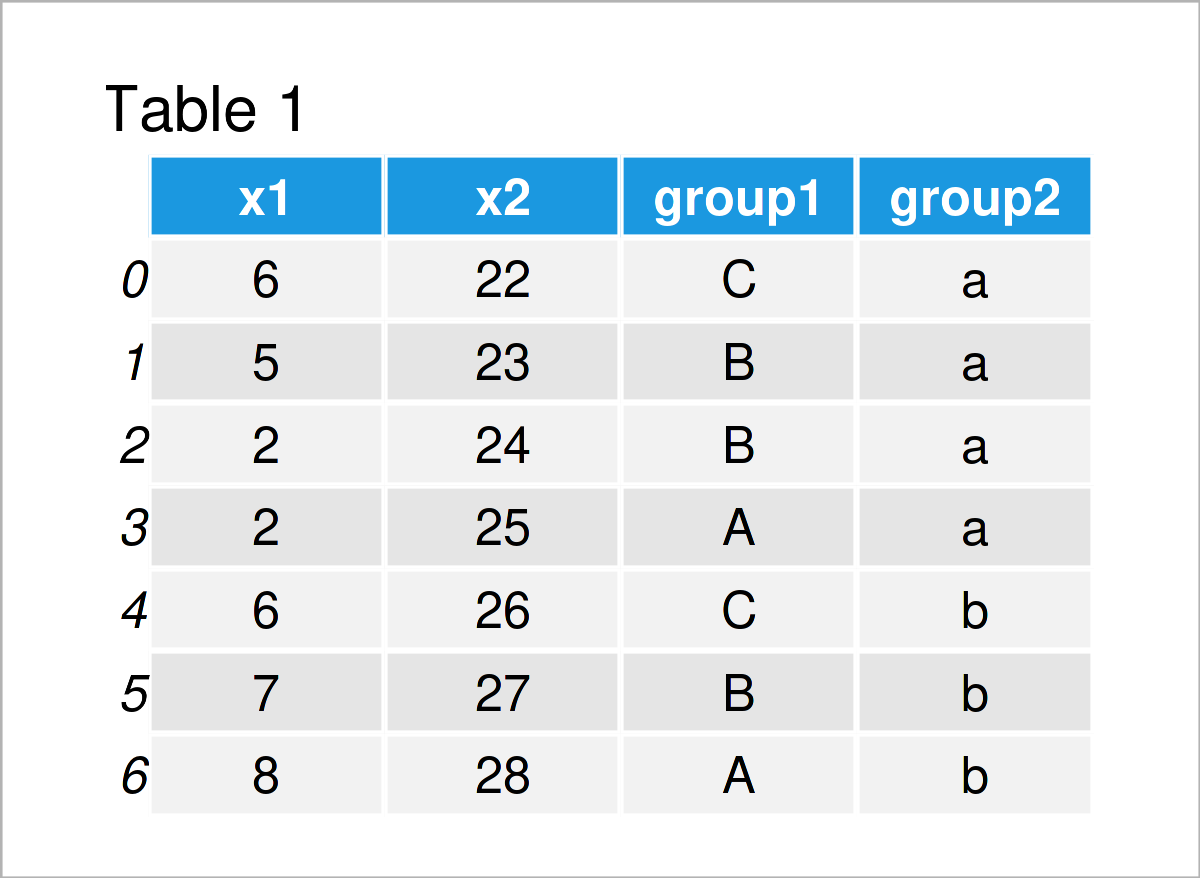# Calculate Sum by Group in Python (2 Examples)

In this article you’ll learn how to compute the sum by group in a pandas DataFrame in the Python programming language.

Let’s dive into it…

## Example Data & Libraries

In order to use the functions of the pandas library, we first need to import pandas:

`import pandas as pd                               # Import pandas`

Let’s also construct an example pandas DataFrame in Python:

```data = pd.DataFrame({'x1':[6, 5, 2, 2, 6, 7, 8],  # Create pandas DataFrame
'x2':range(22, 29),
'group1':['C', 'B', 'B', 'A', 'C', 'B', 'A'],
'group2':['a', 'a', 'a', 'a', 'b', 'b', 'b']})
print(data)                                       # Print pandas DataFrame```Table 1 shows that our example data contains seven rows and four columns. The variables x1 and x2 contain float values and the variables group1 and group2 are our group and subgroup indicators.

## Example 1: Sum by Group in pandas DataFrame

The following Python programming code demonstrates how to take the sum of the values in a pandas DataFrame by group.

To do this, we have to use the groupby and sum functions as shown below:

```print(data.groupby('group1').sum())               # Get sum by group
#         x1  x2
# group1
# A       10  53
# B       14  74
# C       12  48```

The previous output shows the sum of each group and each column separately.

## Example 2: Sum by Group & Subgroup in pandas DataFrame

In this example, I’ll explain how to get the sum for each group and subgroup using two group indicator columns.

For this, we have to specify a list of group variables within the groupby function:

```print(data.groupby(['group1', 'group2']).sum())   # Get sum by multiple groups
#                x1  x2
# group1 group2
# A      a        2  25
#        b        8  28
# B      a        7  47
#        b        7  27
# C      a        6  22
#        b        6  26```

The previous syntax has returned the sum for each subgroup in our DataFrame columns.

## Video & Further Resources

Some time ago, I have released a video on value addition by group on my YouTube channel, which demonstrates the content of this tutorial. You can find the video instruction below:

Please accept YouTube cookies to play this video. By accepting you will be accessing content from YouTube, a service provided by an external third party.If you accept this notice, your choice will be saved and the page will refresh.

Besides that, you might want to have a look at the related articles on my homepage.

Summary: In this article you have learned how to calculate and find the sum by group in the Python programming language. If you have any further questions and/or comments, let me know in the comments.

Subscribe to the Statistics Globe Newsletter